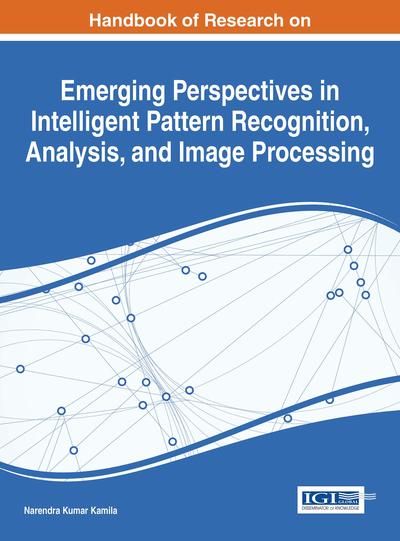# Total Variation Applications in Computer Vision

Vania Vieira Estrela (Universidade Federal Fluminense, Brazil), Hermes Aguiar Magalhães (Universidade Federal de Minas Gerais, Brazil) and Osamu Saotome (InstitutoTecnologico de Aeronautica, Brazil)
DOI: 10.4018/978-1-4666-8654-0.ch002

## Abstract

The objectives of this chapter are: (i) to introduce a concise overview of regularization; (ii) to define and to explain the role of a particular type of regularization called total variation norm (TV-norm) in computer vision tasks; (iii) to set up a brief discussion on the mathematical background of TV methods; and (iv) to establish a relationship between models and a few existing methods to solve problems cast as TV-norm. For the most part, image-processing algorithms blur the edges of the estimated images, however TV regularization preserves the edges with no prior information on the observed and the original images. The regularization scalar parameter ? controls the amount of regularization allowed and it is essential to obtain a high-quality regularized output. A wide-ranging review of several ways to put into practice TV regularization as well as its advantages and limitations are discussed.
Chapter Preview
Top

## 1. Introduction

This chapter investigates robustness properties of machine learning (ML) methods based on convex risk minimization applied to computer vision. Kernel regression, support vector machines (SVMs), and least squares (LS) can be regarded as special cases of ML. The minimization of a regularized empirical risk based on convex functionals has an essential role in statistical learning theory (Vapnik, 1995), because (i) such classifiers are generally consistent under weak conditions; and (ii) robust statistics investigate the impact of data deviations on the results of estimation, testing or prediction methods.

In practice, one has to apply ML methods - which are nonparametric tools - to a data set with a finite sample size. Even so, the robustness issue is important, because the assumption that all data points were independently generated by the same distribution can be contravened and outliers habitually occur in real data sets.

The real use of regularized learning methods depends significantly on the option to put together intelligent models fast and successfully, besides calling for efficient optimization methods. Many ML algorithms involve the ability to compare two objects by means of the similarity or distance between them. In many cases, existing distance or similarity functions such as the Euclidean distance are enough. However, some problems require more appropriate metrics. For instance, since the Euclidean distance uses of the L2-norm, it is likely to perform scantily in the presence of outliers. The Mahalanobis distance is a straightforward and all-purpose method that subjects data to a linear transformation. Notwithstanding, Mahalanobis distances have two key problems: 1) the parameter vector to be learned increases quadratically as data grows, which poses a problem related to dimensionality; and 2) learning a linear transformation is not sufficient for data sets with nonlinear decision boundaries.

Models can also be selected by means of regularization methods, that is, they are penalizing depending on the number of parameters (Alpaydin, 2004; Fromont, 2007). Generally, Bayesian learning techniques make use of knowledge on the prior probability distributions in order to assign lower probabilities to models that are more complicated. Some popular model selection techniques are the Akaike information criterion (AIC), the Takeuchi information criterion (TIC), the Bayesian information criterion (BIC), the cross-validation technique (CV), and the minimum description length (MDL).

This chapter aims at showing how Total Variation (TV) regularization can be practically implemented in order to solve several computer vision applications although is still a subject under research. Initially, TV has been introduced in (Rudin, Osher, & Fatemi, 1992) and, since then, it has found several applications in computer vision such as image restoration (Rudin & Osher, 1994), image denoising (Matteos, Molina & Katsaggelos, 2005; Molina, Vega & Katsaggelos, 2007), blind deconvolution (Chan & Wong, 1998), resolution enhancement (Guichard & Malgouyres, 1998), compression (Alter, Durand, & Froment, 2005), motion estimation (Drulea & Nedevschi, 2011), texture segmentation/discrimination (Roudenko, 2004). These applications involve the use of TV regularization that allows selecting the best solution from a set of several possible ones.

## Key Terms in this Chapter

Variational Method: It is a field of mathematical analysis that works with the maximization or minimization of functionals.

Regularization: It refers to the procedure of bringing in additional knowledge to solve an ill-posed problem or to avoid overfitting. This information appears habitually as a penalty term for complexity, such as constraints for smoothness or bounds on the norm.

Machine Learning: It is concerned with the study of pattern recognition as well as computational learning in artificial intelligence, exploring the structure and studying algorithms that can infer knowledge from and formulate predictions about data. Such algorithms work by building a model from known inputs so as to craft data-driven predictions or decisions, instead of pursuing a predetermined program.

Support Vector Machine: It is concerned with supervised learning models that rely on associated learning algorithms to examine data and to identify patterns, intended for classification, clustering and regression analysis.

Total Least Squares: It is a type of errors-in-variables regression, that is, a least-squares data modeling method in which observational errors on both dependent and independent variables are taken into account. It is basically identical to the best, in the Frobenius norm sense, low-rank approximation of a data matrix.

Total Variation: It is a norm characterized on the space of measures of bounded variation.

Blind Deconvolution: It refers to the implementation of a deconvolution with no explicit information on the impulse response function employed by the related convolution.

## Complete Chapter List

Search this Book:
Reset